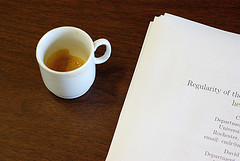# Research

## Overview#### Algebra and Number Theory

Gauss famously referred to the theory of numbers as the “Queen of Mathematics”. It is certainly one of the oldest and most beautiful branches of mathematics, and almost unique with respect to the wide array of tools it uses from subjects such as analysis, algebra and topology. This makes number theory both an extraordinarily rich and challenging field for research.

Rochester has a large, active research group working across the many subfields of number theory including algebraic number theory, analytic number theory, p-adic arithmetic, p-adic cohomology, function field arithmetic, modular forms, the theory of L-functions, Diophantine geometry, and arithmetic dynamics.

#### Analysis

The department has experts in both differential equations and harmonic analysis. Analysis is one of the oldest and broadest areas of mathematics. It contains classical topics, familiar to most college students, such as the theories of differentiation, integration and limits. Applications and connections to other fields, both within and outside mathematics, are numerous.

The Rochester analysis group specializes mainly in harmonic analysis and partial differential equations. Harmonic analysis studies how functions can be broken up into basic waves and applications thereof. The Fourier transform and Fourier series are examples of topics included in harmonic analysis. Applications are extensive in such fields as signal processing, medical imaging and quantum mechanics.

Partial differential equations are equations that are frequently used to model real world problems. Understanding the solutions, such as whether they exist, are unique and how they behave in general, is the primary interest. Such equations play a prominent role in physics, engineering, economics and other disciplines. Partial differential equations are a rich source of problems in harmonic analysis.

#### Combinatorics

The combinatorics group is interested in intersections of combinatorics with other areas of mathematics such as analysis, algebra, topology, geometry and number theory.

#### Geometry

The geometry group works in a number of different areas. Geometry has been one of the most popular fields of mathematics in recent decades. It combines techniques from apparently unconnected areas, such as the use of analytical tools for answering topological questions, and it has strong relations with quantum field theory and string theory.

Recent results in geometry include the proof of the Poincare conjecture, by G. Perelman in 2002-2003 following the analytic program of R. Hamilton, and the differentiable sphere theorem, proved by S. Brendle and R. Schoen in 2008-2009.

#### Probability, Ergodic Theory, and Mathematical Physics

In recent years probability has blossomed. Interactions with other fields such as biology, computer science, finance, and especially statistical physics have led to a broadening and deepening of the subject.

Although probability began in the early 1600s, the subject as we know it today dates from the twentieth century, when powerful tools from abstract analysis brough new rigor to the field. As scientists study nature in ever greater detail, they increasingly find that random effects play an essential role in almost all descriptions of natural phenomena. These new insights enriched probability, and spilled over into many other parts of mathematics.

In addition to probabilists, there are several associated faculty members in mathematical physics.

#### Topology

The department has experts in algebraic topology, particularly in homotopy theory. The 20th century has been called the century of topology. General topology now forms one of the foundations of modern analysis and geometry.

The development of algebraic topology led to the development of many of the key concepts of algebra: homological algebra, category theory, Lie groups/algebras and K-theory among them. The use of cobordism invariants and intersection theory developed in differential topology form the foundations for invariants such as the Seiberg-Witten invariants and Gromov-Witten invariants so important in current day geometry.

Today topology continues to flourish as a pure endeavor. Symplectic topology and Voevodsky’s recent introduction of the techniques of homotopy theory into algebraic geometry are among some examples. In addition, there are growing connections with theoretical physics, dynamical systems, computer science and DNA biology.